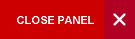## ECON3031: PROBABILITY AND DISTRIBUTION THEORY FOR BUSINESS AND SOCIAL SCIENCES

Outline: Concepts (classical probability, mathematical and empirical concepts of probability in terms of set theory concepts of events (mutually exclusive, conditional, independent etc.), axioms and rules of probability - Baye's theorem etc. Mathematical expectation (random variables, expectations, probability frequency function, probability density functions, joint density function, moments and moment generating function, Chebysheff's theorem, law of large numbers etc.); Probability distributions (discrete uniform distribution, binomial distribution, hypergeometric distribution, family of exponential distributions, normal distribution and normal approximation to the binomial distribution etc.); sampling distributions t, chi-square and F distributions - applications).

Pre-requisites: ECON2008 or STAT2001 & ECON1004 or MATH1142 (Anti-requisite: MATH2404)

Semester: 1

Lecturer: Ms. Romae Finegan-Muschette

Position: Assistant Lecturer

Office Hours: N/A

Office Location: (Sir Alister McIntyre Building), Block E E-205